Jet Set Go! All about Aeroplanes Jet Set Go! All about Aeroplanes

# Class 10 Maths Chapter 9 Some Applications of Trigonometry MCQs

Class 10 Maths Chapter 9 MCQs (Some Applications of Trigonometry) are available online here at BYJU’S with solved answers. All the objective questions are prepared as per the CBSE board syllabus (2022 – 2023) and NCERT guidelines. Practising these questions will help students to score good marks in the board exam. Also, click here to get chapter-wise multiple-choice questions.

Also, check: Heights and Distances (An application of trigonometry)

## Class 10 Maths Chapter 9 MCQs (Some Applications of Trigonometry)

Solve the questions below for Chapter 9 (Some applications of trigonometry) and increase your chances of scoring high in this chapter. Also, get important questions for class 10 Maths here to practice more.

Students can also get access to Some Applications of Trigonometry Class 10 Notes here.

1. If the length of the shadow of a tree is decreasing then the angle of elevation is:

(a) Increasing

(b) Decreasing

(c) Remains the same

(d) None of the above

Explanation: See the following figure:As the shadow reaches from point D to C towards the direction of the tree, the angle of elevation increase from 30° to 60°.

2. The angle of elevation of the top of a building from a point on the ground, which is 30 m away from the foot of the building, is 30°. The height of the building is:

(a) 10 m

(b) 30/√3 m

(c) √3/10 m

(d) 30 m

Explanation: Say x is the height of the building.

a is a point 30 m away from the foot of the building.

Here, height is the perpendicular and distance between point a and foot of building is the base.

The angle of elevation formed is 30°.

Hence, tan 30° = perpendicular/base = x/30

1/√3 = x/30

x = 30/√3

3. If the height of the building and distance from the building foot’s to a point is increased by 20%, then the angle of elevation on the top of the building:

(a) Increases

(b) Decreases

(c) Do not change

(d) None of the above

Explanation: We know, for an angle of elevation θ,

tan θ = Height of building/Distance from the point

If we increase both the value of the angle of elevation remains unchanged.

4. If a tower 6m high casts a shadow of 2√3 m long on the ground, then the sun’s elevation is:

(a) 60°

(b) 45°

(c) 30°

(d) 90°

Explanation: As per the given question: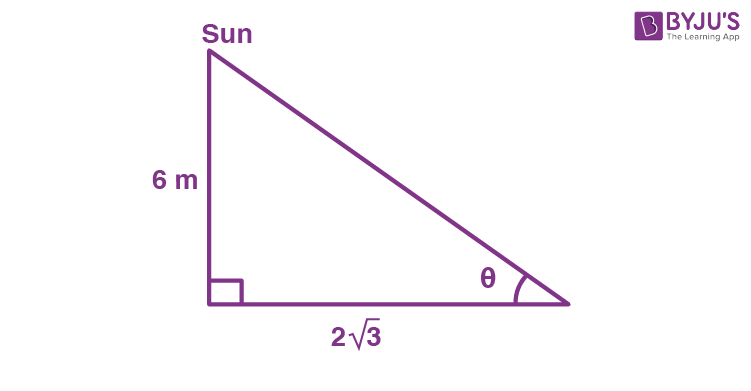Hence,

tan θ = 6/2√3

tan θ = √3

tan θ = tan 60°

⇒ θ = 60°

5. The angle of elevation of the top of a building 30 m high from the foot of another building in the same plane is 60°, and also the angle of elevation of the top of the second tower from the foot of the first tower is 30°, then the distance between the two buildings is:

(a) 10√3 m

(b) 15√3 m

(c) 12√3 m

(d) 36 m

Explanation: As per the given question: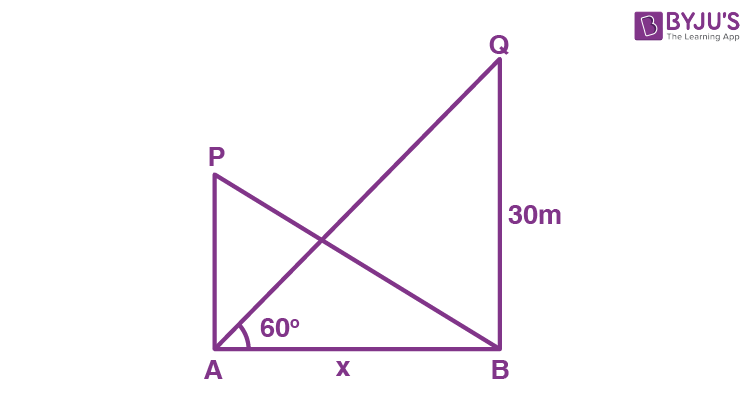Hence,

tan 60° = 30/x

√3 = 30/x

x = 30/√3

x = 10√3m

6. The angle formed by the line of sight with the horizontal when the point is below the horizontal level is called:

(a) Angle of elevation

(b) Angle of depression

(c) No such angle is formed

(d) None of the above

The angle formed by the line of sight with the horizontal when the point is below the horizontal level is called angle of depression.

7. The angle formed by the line of sight with the horizontal when the point being viewed is above the horizontal level is called:

(a) Angle of elevation

(b) Angle of depression

(c) No such angle is formed

(d) None of the above

The angle formed by the line of sight with the horizontal when the point being viewed is above the horizontal level is called angle of elevation.

8. From a point on the ground, which is 15 m away from the foot of the tower, the angle of elevation of the top of the tower is found to be 60°. The height of the tower (in m) standing straight is:

(a) 15√3

(b) 10√3

(c) 12√3

(d) 20√3

Explanation: We know:

tan (angle of elevation) = height of tower/its distance from the point

tan 60° = h/15

√3 = h/15

h = 15√3

9. The line drawn from the eye of an observer to the point in the object viewed by the observer is said to be

(a) Angle of elevation

(b) Angle of depression

(c) Line of sight

(d) None of the above

The line drawn from the eye of an observer to the point in the object viewed by the observer is said to be line of sight.

10. The height or length of an object or the distance between two distant objects can be determined with the help of:

(a) Trigonometry angles

(b) Trigonometry ratios

(c) Trigonometry identities

(d) None of the above

The height or length of an object or the distance between two distant objects can be determined with the help of trigonometry ratios.

11. When the shadow of a pole h metres high is √3h metres long, the angle of elevation of the Sun is

(a) 30°

(b) 60°

(c) 45°

(d) 15°

Explanation:

Let AB be the pole and BC be its shadow.

Consider θ is the angle of elevation of the Sun.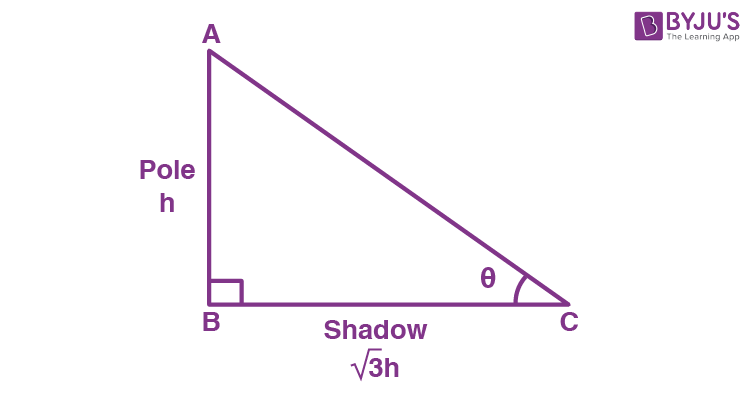In right triangle ABC,

tan θ = AB/BC = h/√3h = 1/√3

tan  θ = tan 30°

θ = 30°

12. A ladder makes an angle of 60° with the ground, when placed along a wall. If the foot of ladder is 8 m away from the wall, the length of ladder is

(a) 4 m

(b) 8 m

(c) 8√3 m

(d) 16 m

Explanation:

Let AB be the wall, AC be the length of the ladder.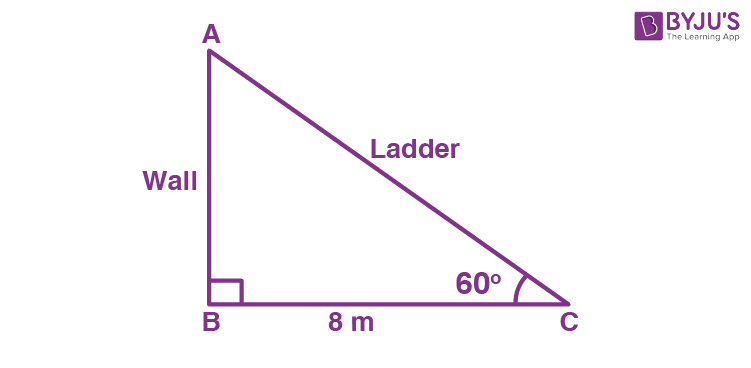In right angled triangle ABC,

cos 60° = BC/AC

½ = 8/AC

AC = 8 × 2 = 16

Therefore, the length of the ladder is 16 m.

13. If the height and length of a shadow of a tower are the same, then the angle of elevation of Sun is

(a) 30°

(b) 60°

(c) 45°

(d) 15°

Explanation:

Let AB be the tower and BC be its shadow.

Consider the angle of elevation of the Sun as θ.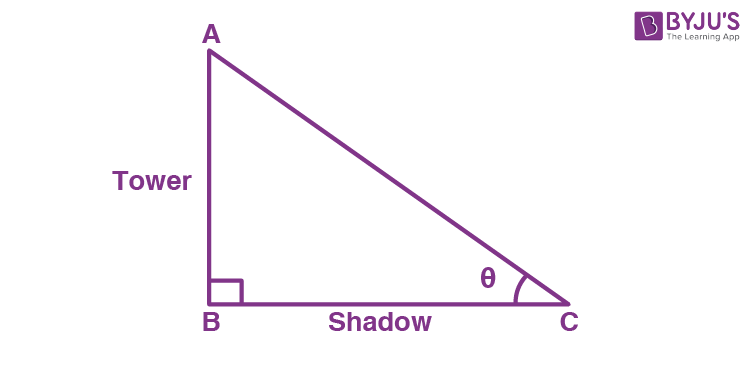According to the given,

AB = BC

In right triangle ABC,

tan θ = AB/BC

tan θ = AB/AB {since AB = BC}

tan θ = 1

tan θ = tan 45°

θ = 45°

14. The angle of depression of an object on the ground, from the top of a 25 m high tower is 30°. The distance of the object from the base of tower is

(a) 25√3 m

(b) 50√3 m

(c) 75√3 m

(d) 50 m

Explanation:

Let AB be the tower and BC be the distance of the object (at C) from the base of the tower.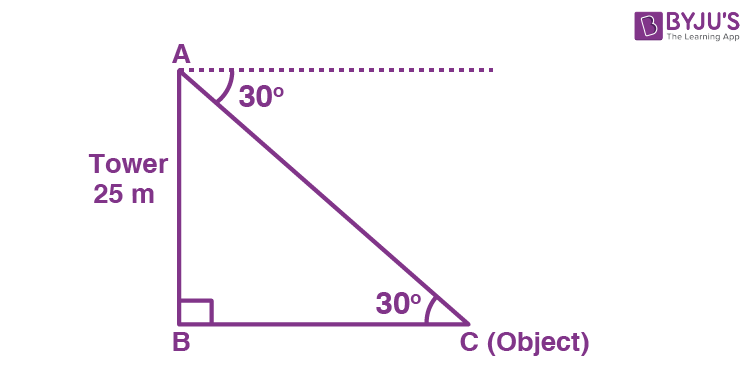In right triangle ABC,

tan 30° = AB/BC

1/√3 = 25/BC

BC = 25√3 m

15. The shadow of a tower standing on level ground is found to be 40 m longer when the Sun’s altitude is 30° than when it was 60°. The height of the tower is

(a) 40√3 m

(b) 20√3

(c) 20 m

(d) 15√3 m

Explanation:

When the sun’s altitude is the angle of elevation of the top of the tower from the tip of the shadow.

Let AB be h m and BC be x m. From the question, DC is 40 m longer than BC.

So, BD = (40 + x) m

And two right triangles ABC and ABD are formed as shown in the figure.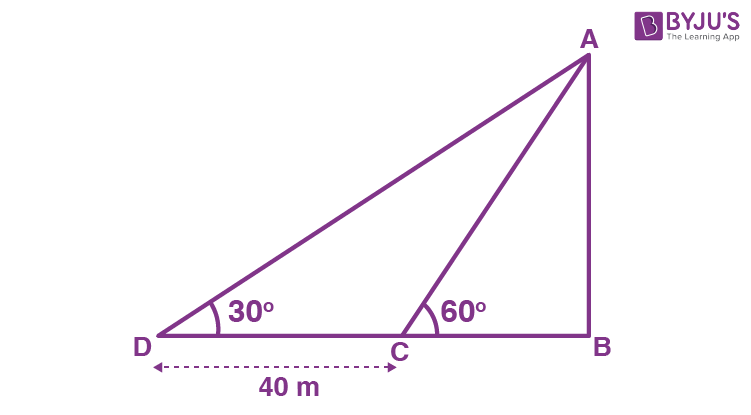In ΔABC,

tan 60° = AB/ BC

√3 = h/x

x = h/√3….(i)

In ΔABD,

tan 30° = AB/ BD

1/ √3 = h/ (x + 40)

x + 40 = √3h

h/√3 + 40 = √3h [using (i)]

h + 40√3 = 3h

2h = 40√3

h = 20√3

Therefore, the height of the tower is 20√3 m.

16. If the angles of elevation of the top of a tower from two points at the distance of a m and b m from the base of tower and in the same straight line with it are complementary, then the height of the tower (in m) is

(a) √(a/b)

(b) √ab

(c) √(a + b)

(d) √(a – b)

Explanation:

If the angles of elevation of the top of a tower from two points at the distance of a m and b m from the base of tower and in the same straight line with it are complementary, then the height of the tower (in m) is √ab.

17. From a point on a bridge across a river the angle of depression of the banks on opposite sides of the river are 30° and 45° respectively. If the bridge is at the height of 30 m from the banks, the width of the river is

(a) 30(1 + √3) m

(b) 30(√3 – 1) m

(c) 30√3 m

(d) 60√3 m

Answer: (a) 30(1 + √3) m

Explanation:

The bridge is at a height of 30 m from the banks.

Let, A and B represent the points on the bank on opposite sides of the river. And, AB is the width of the river. P is a point on the bridge which is at the height of 30 m from the banks.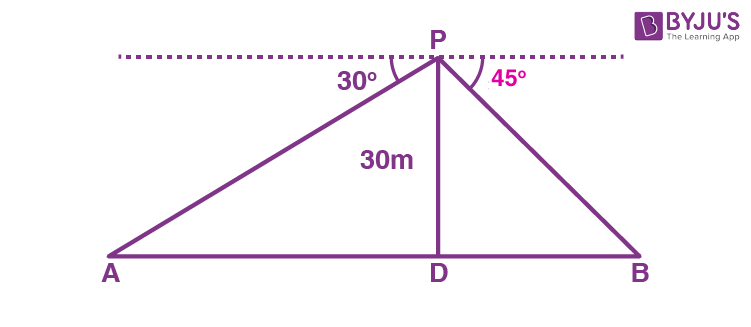In right ΔAPD,

∠A = 30°

So, tan 30° = PD/ AD

Next, in right ΔPBD

∠B = 45°

So, tan 45° = PD/ BD

1 = PD/ BD

BD = PD

BD = 30 m

We know that, AB = AD + DB = 30√3 + 30 = 30(√3 + 1)

Hence, the width of the river = 30(1 + √3)m

18. The ratio of the height of a tower and the length of its shadow on the ground is √3 : 1. The angle of elevation of the Sun is

(a) 30°

(b) 45°

(c) 60°

(d) 75°

Explanation:

Let AB be the height and BC be its shadow.

θ be the angle of elevation of the Sun.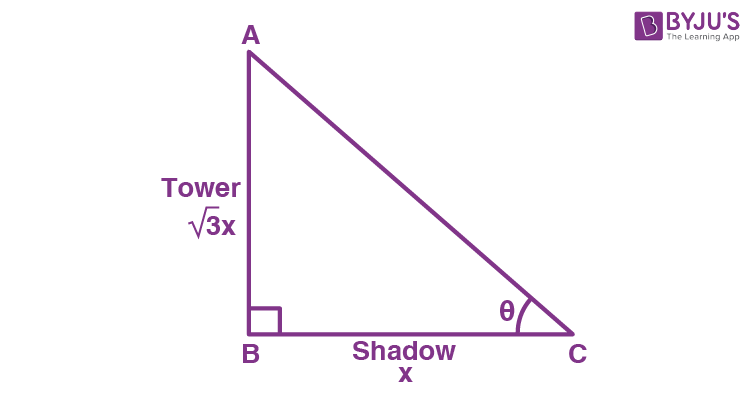According to the given, AB : BC = √3 : 1

So, AB = √3x and BC = x

In right triangle ABC,

tan θ = AB/BC

tan θ = √3x/x

tan θ = √3

tan θ = tan 60°

θ = 60°

19. A tree breaks due to a storm and the broken part bends so that the top of the tree touches the ground making an angle of 30° with the ground. The distance between the foot of the tree to the point where the top touches the ground is 8 m. The height of the tree is

(a) 4√3 m

(b) 8√3 m

(c) 6√3 m

(d) 16√3 m

Explanation:

Let AC be the initial height of the tree.

Due to the storm the tree is broken at B.

Let the bent portion of the tree be AB = x m and the remaining portion BC = h m.

So, the height of the tree AC = (x + h) m

And, given DC = 8m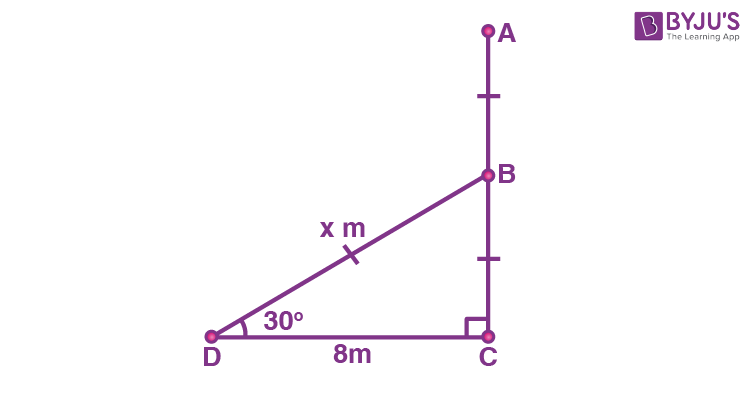In right ΔBCD,

tan 30° = BC/DC

1/√3 = h/8

h = 8/√3

Again in right ΔBCD,

cos 30° = DC/BD

√3/2 = 8/x

x = 16/√3 m

So, x + h = 16/√3 + 8/√3

= 24/√3

= (24 . √3)/(√3 . √3)

= (24√3)/3

= 8√3

Therefore, the height of the tree is 8√3 m.

20. The angle of elevation of the top of a tower is 30°. If the height of the tower is doubled, then the angle of elevation of its top will be

(a) Greater than 60°

(b) Equal to 30°

(c) Less than 60°

(d) Equal to 60°

Explanation:

Let AB = h be the height and BC = x.

In this case, the angle of elevation is 30°.

When height is doubled, the height of the tower will be PQ = 2h.

In this case, consider the angle of elevation as θ.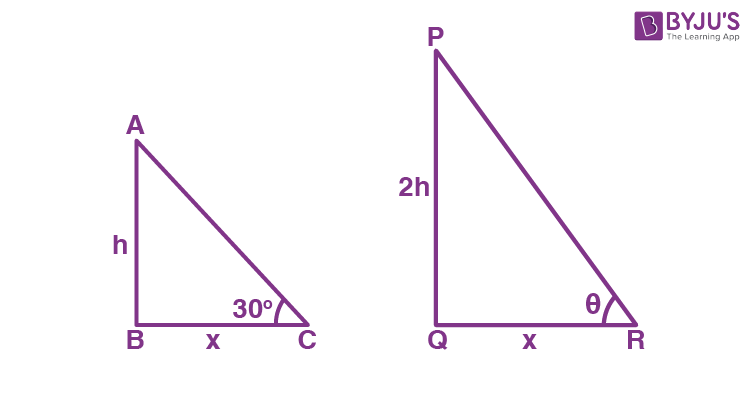Also, BC = QR = x.

In triangle ABC,

tan 30° = AB/BC = h/x

1/√3 = h/x….(i)

In triangle PQR,

tan θ = PQ/QR

tan θ = 2h/x

tan θ = 2(1/√3) {from (i)}

tan θ = 1.15 (approx)

θ = tan-1(1.15) < 60°.

### Video Lesson on Trigonometry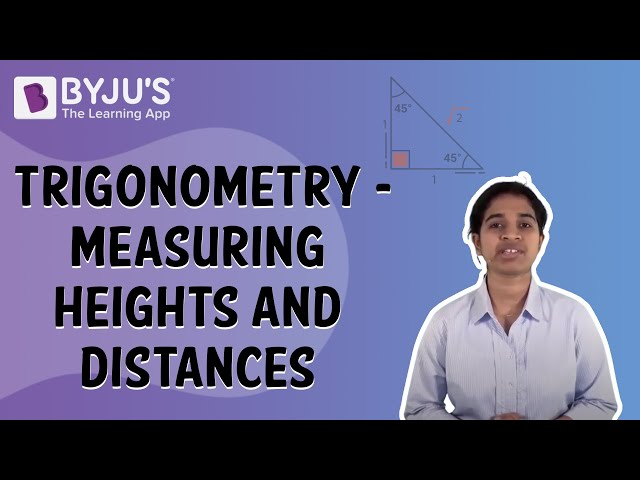Stay tuned with BYJU’S – The Learning App to get all chapter-wise MCQs for class 10 Maths and learn all Maths-related concepts quickly by exploring more videos.